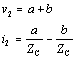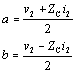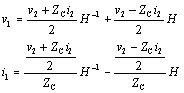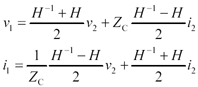# Simple Cases Involving Transmission Lines

Figure C.3 summarizes three classic forms of transmission matrices used to define the overall system gain of a typical transmission-line configuration. In the figure Z C represents the characteristic impedance and H the one-way transfer function of a transmission line.

Figure C.3. These three forms of transmission matrix are often used to describe digital transmission circuits.The derivations of the top two forms in Figure C.3 are self-evident from the definition of the transmission matrix (Figure C.1). The third form corresponding to a transmission line is developed in the following way.

The general form of solution for the signals on a transmission line is composed of two traveling waves, one propagating to the right and one moving to the left (see Section 2.2.5, Figure 2.6). Suppose that at the right-hand end of the line the signal amplitudes of the two waves are denoted a and b . The currents associated with these two waveforms at that point must then be + a/Z C (a current moving to the right) and - b / Z C (representing a current moving to the left). At the right-hand end of the line, the superposition of these waves must generate the voltage and current extant at that end.

Equation C.4The preceding conditions may be inverted to determine the amplitudes a and b .

Equation C.5At the left end of the line the same conditions prevail, except that the amplitudes of the right- and left-traveling waveforms must be adjusted to account for their propagation through the transmission medium. The amplitude of the left-going waveform is diminished by H , the one-way transfer function of the transmission line, while the amplitude of the right-traveling waveform must be increased by H “1 , so that after traveling to the right end of the line, it will appear at the correct amplitude a . Summing the voltages and currents at the left end of the line produces a relationship between a , b and v 1 , i 1 .

Equation C.6Now substitute for a and b the relations to v 2 and i 2 .

Equation C.7Collecting together the terms associated with v 2 and i 2 respectively reveals the form of the transmission matrix.

Equation C.8High-Speed Signal Propagation[c] Advanced Black Magic
ISBN: 013084408X
EAN: N/A
Year: 2005
Pages: 163